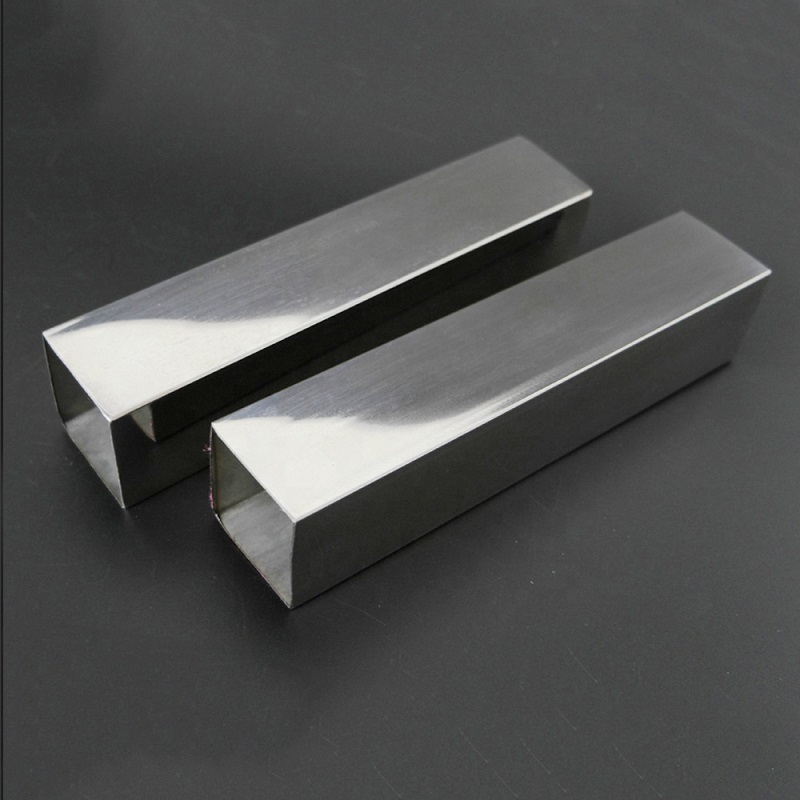# how much weight can square steel tubing hold

For any steel pipe, there is a bearing capacity, and the house pipe is no exception. Strength refers to the performance of metal materials to resist damage (excessive plastic deformation or fracture) under static load. Since the load acts in the form of tension, compression, bending, shearing, etc., the strength is also divided into tensile strength, compressive strength, bending strength, and shear strength. There is often a certain relationship between various strengths, and tensile strength is generally used as the most basic strength indicator in use.

The bearing capacity of square steel tubing is divided into the following situations:

1. Only tensile force: This is the simplest, the tensile force divided by the cross-sectional area of ​​the square steel tubing is less than the allowable tensile stress of the square steel tube.

2. Shearing force only: This is also simple. The shearing force divided by the cross-sectional area of ​​the square steel pipe is less than the allowable shear stress of the square steel pipe.

3. Only under pressure: not only the pressure divided by the cross-sectional area of ​​the square steel tubing is smaller than the allowable compressive stress of the square steel pipe, but also whether it will lose stability under pressure.

4. Square steel tube bending: not only calculate whether the maximum local stress exceeds the allowable stress, but also consider the moment of inertia of the section and the modulus of elasticity.

5. Torsion of square steel tubing: It is also necessary to calculate whether the maximum local stress exceeds the allowable stress, which is also related to the section moment of inertia and elastic modulus.The calculation method is different according to the force mode:

1) Tension action 2) Shear force action 3) Bending moment action 4) Torsion 5) Multiple load combination action
Finding the stress and deflection deformation is mainly related to the parameter properties of the load and the section, and then find the formula and set the formula. For specific formulas, please refer to the books on mechanics of materials. However, the problem must be clearly described here, such as the force model (it is almost cantilevered), load conditions, component parameters (section, component length), etc., so that we can figure out what formula to use.

The pressure of square steel tubing can be calculated by referring to the following simple formula:

One: The calculation method of knowing the outer diameter and wall thickness of the square steel tube to withstand the pressure (different materials of square steel tube have different tensile strength)

Pressure=(wall thickness*2*tensile strength of square steel pipe material)/(outer diameter*factor)

Two: The method of calculating the wall thickness by knowing the outer diameter of the square steel pipe and the pressure:

Square steel tubing wall thickness=(pressure*outer diameter*factor)/(2*square steel tube material tensile strength)

Three: Expression method of square steel pipe pressure coefficient:

Pressure P<7Mpa coefficient S=8

7<square steel pipe pressure P<17.5 coefficient S=6

Pressure P>17.5 coefficient S=4

For example: the thickness is 10mm, the length is 1144mm, and the cross-sectional area is 200*100. I want to know what is the formula for the bearing capacity of a square tube? What should be calculated?

First of all, you must know:

1. The thickness of the pipe wall, the material of the square steel tubing, the tensile strength, and the pressure coefficient of the steel pipe

2. Whether the force is a column or a beam, whether it is a column or an eccentric force, or whether a beam is a concentrated force or a uniform force.

3. The length of the square tube

It is further understood that the material of the square steel tube is Q345B, the tensile strength is 525, and the steel pipe pressure is 15. Then, the calculation formula is as follows:

The pressure of the square steel tubing = (8*2*525)/(191*6)=9.162.

Sino East Steel supplies high quality and strength 1 inch square tubing,  2 inch galvanized pipe4×4 square steel tubing, etc,. for buyers.